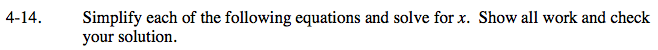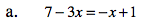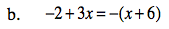### Home > CAAC > Chapter 4 > Lesson 4.1.2 > Problem4-14

4-14.7 + −3x = −x + 1

Now you have to choose!

Subtract 7 from both sides.

Flip to positive.

7 + −2x = 1

−2x = −6

2x = 6

Subtract 1 from both sides.

7 = 2x +1

2x = 6

Divide by 2 on both sides.

x = 3